# Electronics and Communication Engineering - Exam Questions Papers

41.

A λ/4 long high frequency transmission line is terminated into one impedance ZR. If Z0 be the characteristic impedance of the line, then input impedance Zin is

 A. ZoZR B. Z2R/Zo C. Z20/ZR D. Infinity

Explanation: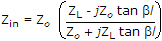, here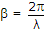and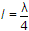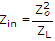[Here ZL = ZR].

42.

Find the power absorbed or delivered by each source: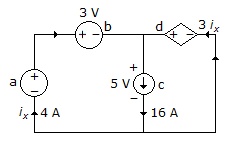A. a = -32 b = - 12 c = -80 d = -60 B. a = -32 b = - 12 c = 80 d = 60 C. a = 32 b = 12 c = -80 d = 60 D. a = -32 b = 12 c = 80 d = -60

Explanation:

Source a : Power : 8 x (4) = - 32 W. Source A has delivered the power as it is. - ve.

Source b: Source B has absorbed power of + 12 W as power is positive

Source c: Source C has absorbed power of 5 x 16 = 80 W

Source d: Delivers power of 3 x ix x (5) = 3 x 4 x (- 5) = -60 W

Total power of the network is - 32 + 12 + 80 - 60 = 0 Watts.

43.

The function shown in figure, can represent a probability density function for A __________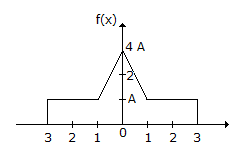A. A = 1/4 B. A = 1/6 C. A = 1/9 D. A = 1/2

Explanation:

The condition for the probability density function d(x) is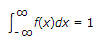and is always non negative or the area under the curve f(x)is unity.

=> A x 6 +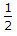x 2 x 3A = 1

or 9A = 1 or A =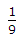.

44.

If the transfer function of the following network is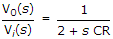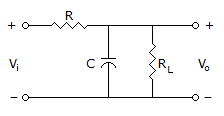The value of the load resistance RL is

 A. R/4 B. R/2 C. R D. 2R

Explanation: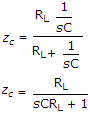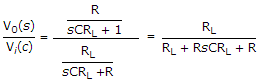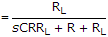.

45.

A bipolar differential amplifier uses a transistor having β0 = 200, ICQ = 100 mA, |Adm| = 500 and CMRR = 80 dB. The value of Rc and RE will be respectively __________ .

 A. 1.25 kΩ, 1.25 MΩ B. 125 kΩ, 1.25 MΩ C. 1.25 MΩ, 1.25 kΩ D. 3.25 kΩ, 3.25 MΩ

Explanation:

80 = 20 log CMRR

CMRR = 10000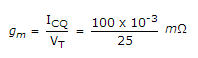But CMRR = 2gmRE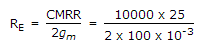= 1.25 MΩ

Adm = - gmRC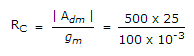= 1.25 KΩ.

#### Current Affairs 2022

Interview Questions and Answers